maths > wholenumbers

Ascending and Descending Orders (Whole Numbers)

what you'll learn...

overview

More than two numbers can be compared to arrange them in

•  ascending order : from smallest to the largest

•  descending order : from largest to the smallest

order in many

the three trees shown in the figure. The pine tree oak tree and the palm tree. The largest among them is placed first position as pine. Then the rest are compared and largest among them is placed in second position as palm. Third position is taken by the last oak tree. Thus, the trees can be ordered from largest to smallest.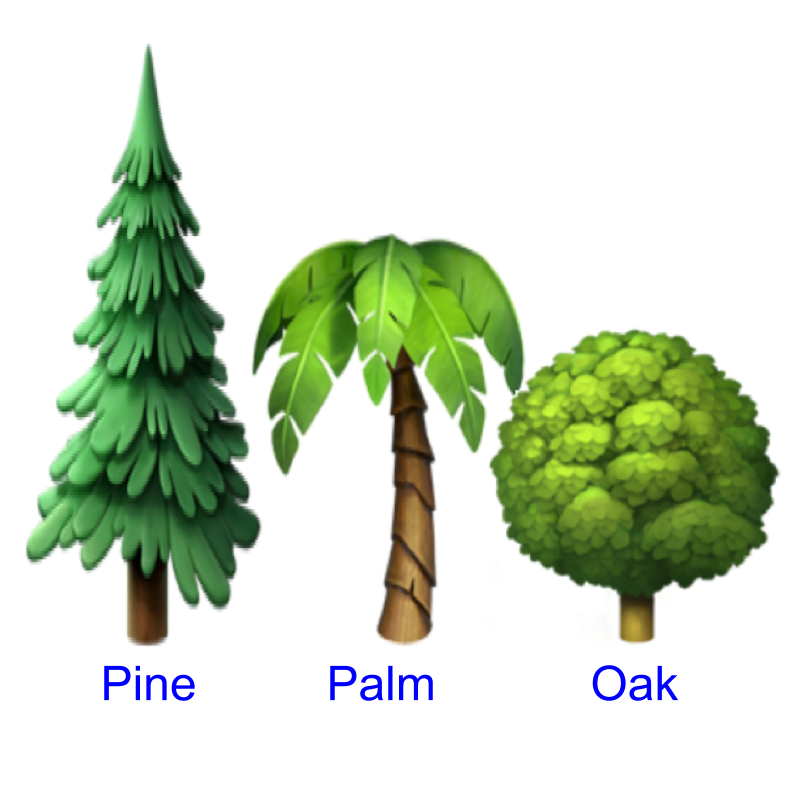Considering the problem : arranging trees from largest to smallest. The order is Pine, Palm, and Oak. The numbers (in this example, representing the height of trees) can be arranged from largest to smallest. This order is called descending order.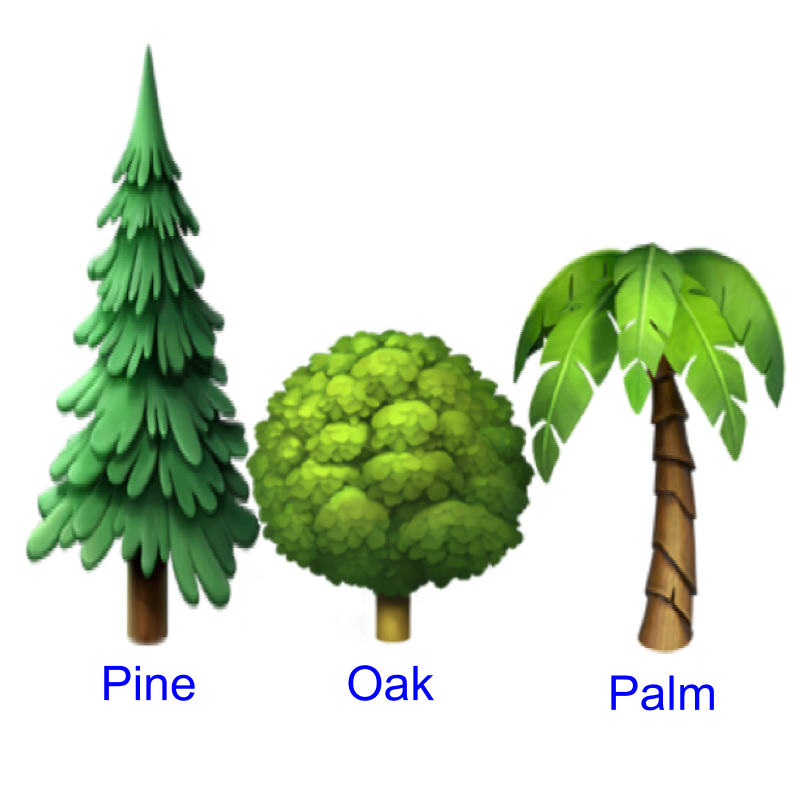The trees are ordered from smallest to largest. The smallest among them is placed first position as oak. Then the rest are compared and smallest among them is placed in second position as palm. Third position is taken by the last pine tree. Thus, the trees are ordered from smallest to largest.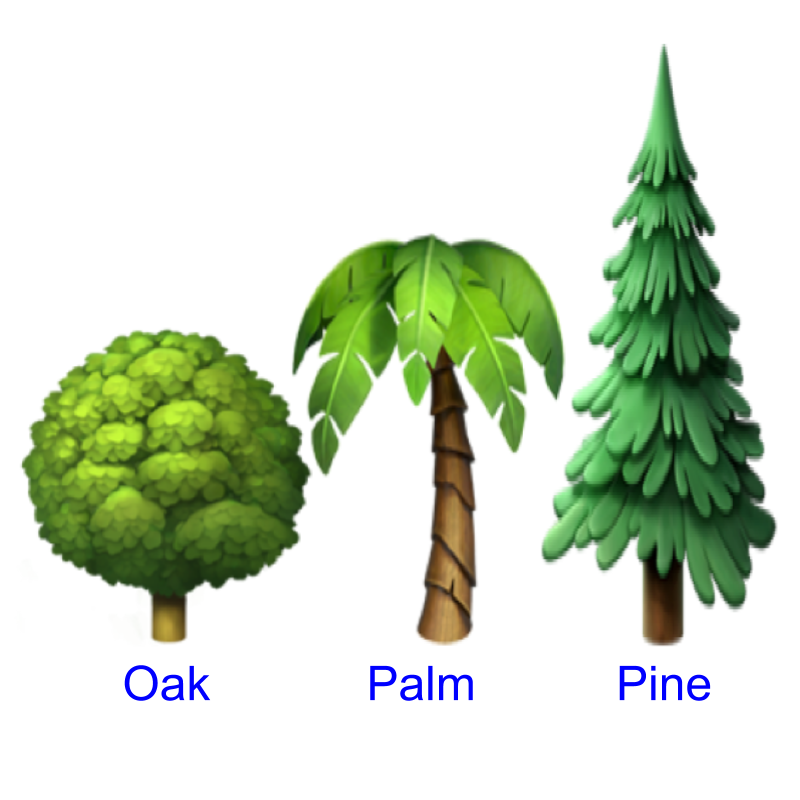The order is Oak, Palm, and Pine. The numbers (in this example, representing the height of trees) can be arranged from smallest to largest. This order is called ascending order.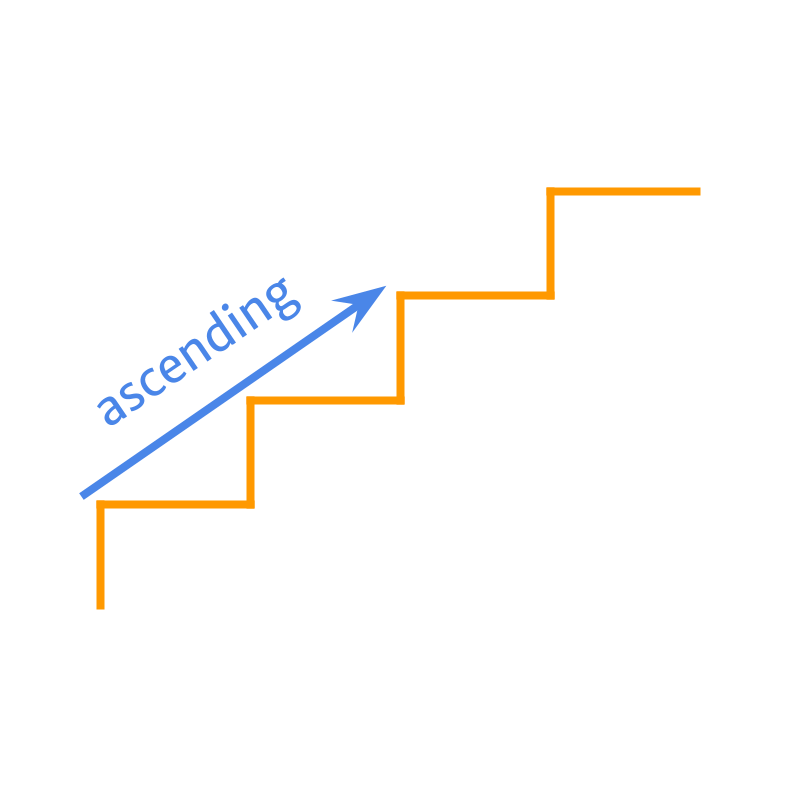The word "ascend" means: to go up or climb. The ascending order : the numbers are ordered from smallest to the largest.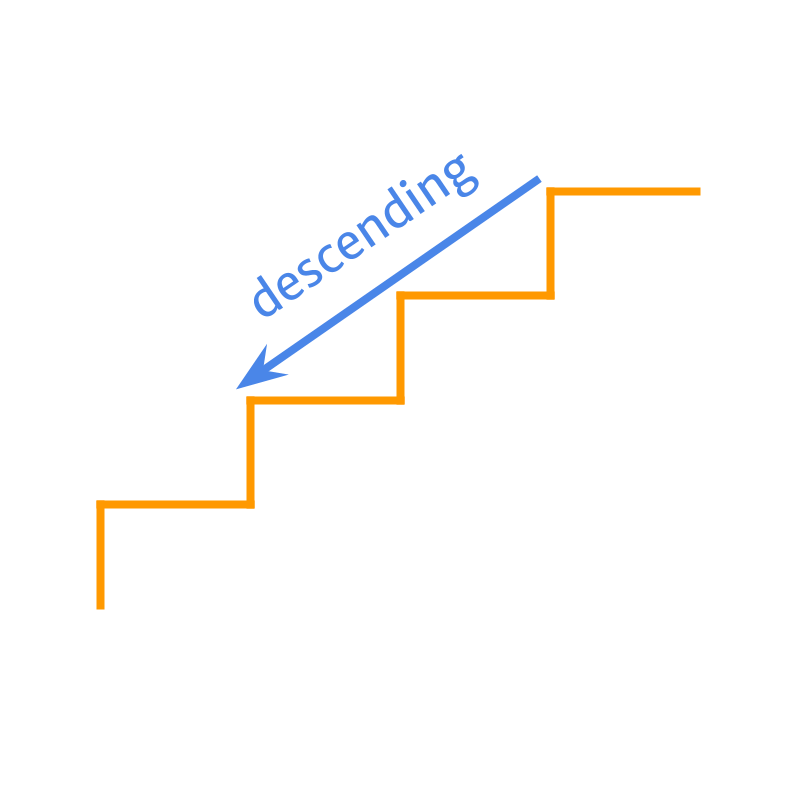The word "descend" means: to move down. The descending order : the numbers are ordered from largest to the smallest.

Arrange the numbers in ascending order 234$234$, 73$73$, 423$423$.
The answer is "73$73$, 234$234$,423$423$"

Arrange the numbers in descending order $873$, $9$, $99$.
The answer is "$873$, $99$, $9$"

More than two numbers can be compared to arrange them in
•  ascending order : from smallest to the largest
•  descending order : from largest to the smallest

examples

Arrange the numbers in descending order $588$, $23$, $21$.
The answer is "$588$, $23$, $21$"

summary

More than two numbers can be compared to arrange them in

•  ascending order : from smallest to the largest•  descending order : from largest to the smallestExample: given numbers $2 , 7 , 4$ is arranged in
ascending order : $2 , 4 , 7$
descending order : $7 , 4 , 2$

Outline